Homework Help Question & Answers

In solving for the damped forced circuit 1 oscillations (particular solution) has the form 6. +R ...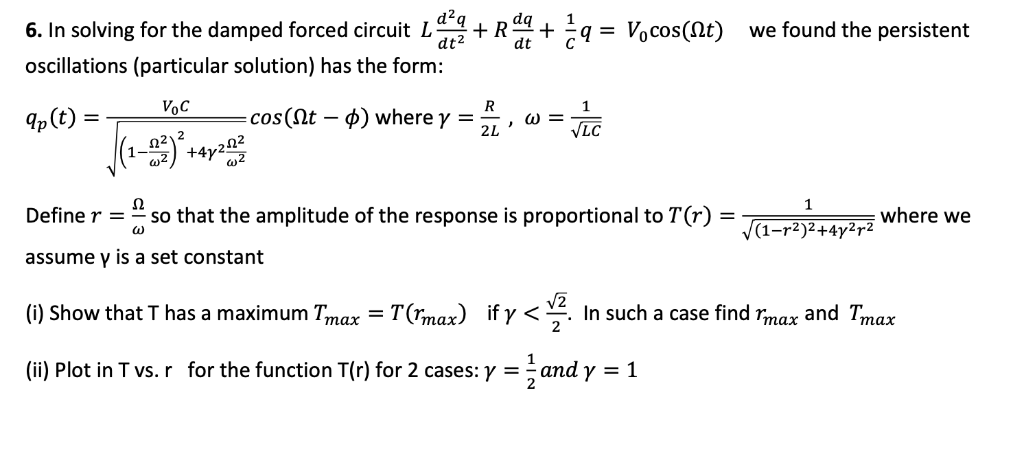In solving for the damped forced circuit 1 oscillations (particular solution) has the form 6. +R q-Vocos(r) + dt C we found the pers istent cos (st where γ -, LC 21 2 Define rso that the amplitude of the response is proportional toT(r)- where we (1-r2)2 +4γ assume γ is a set constant (i) Show that T has a maximum Tmax-T(rmax) if γく1. In such a case find "max and T,nax (ii) Plot in T vs. r for the function T(r) for 2 cases: γ-2 and γ-1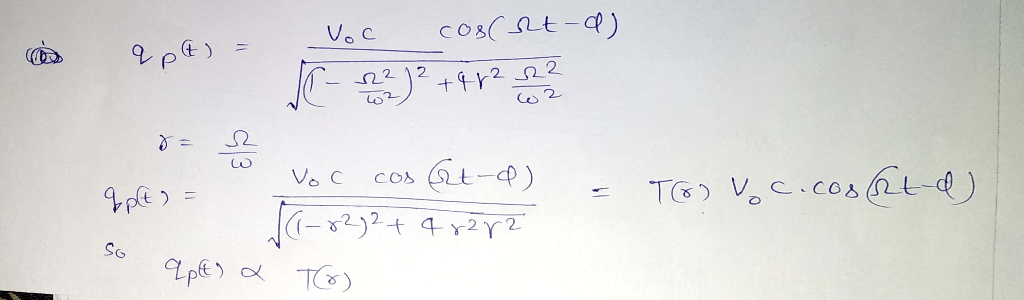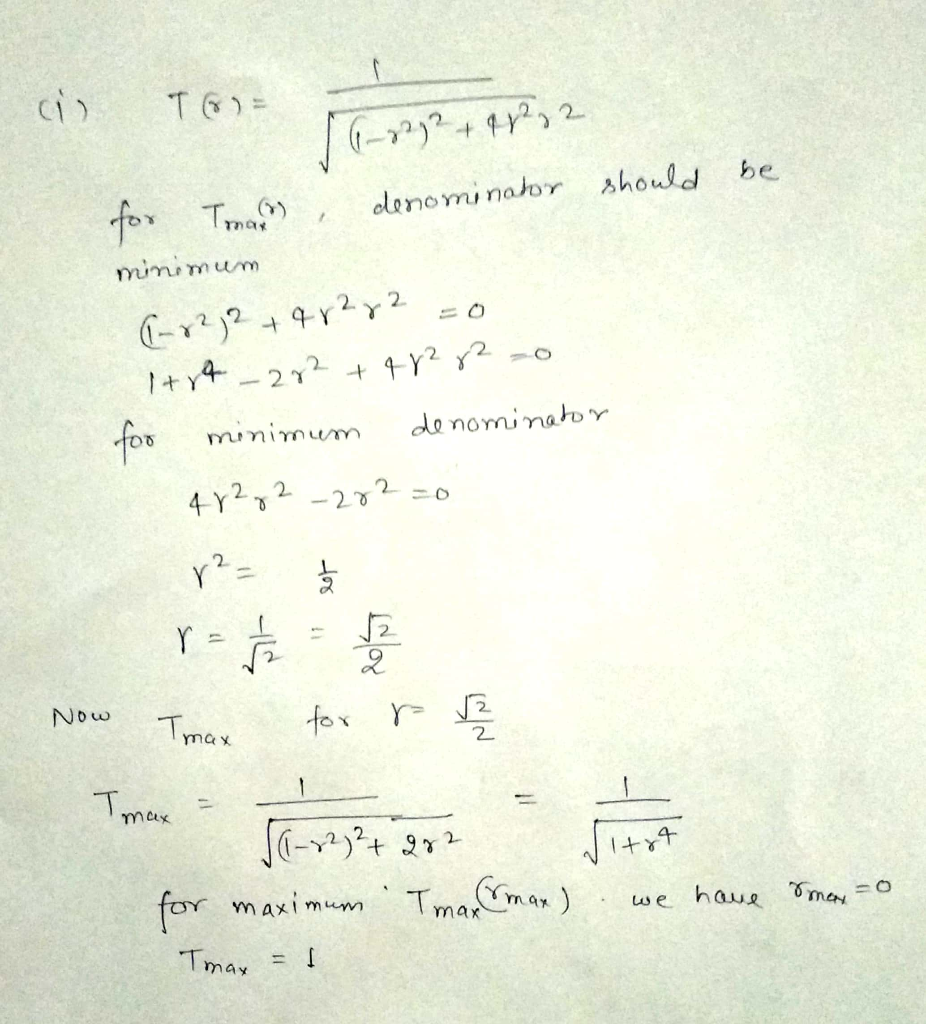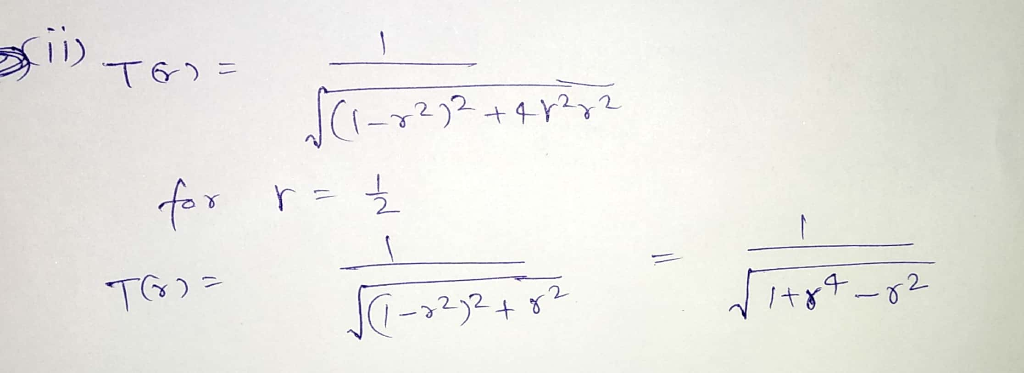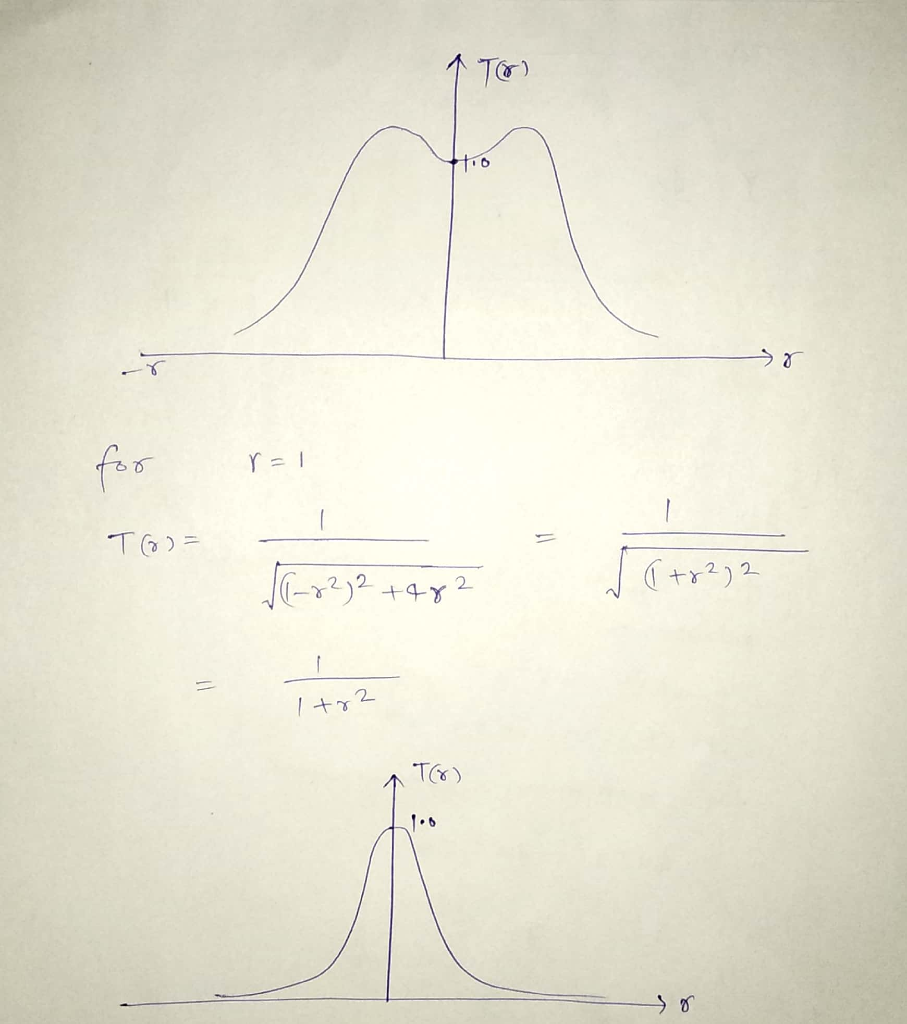Add Answer of: In solving for the damped forced circuit 1 oscillations (particular solution) has the form 6. +R ...
More Homework Help Questions Additional questions in this topic.

• A free-spring system, damped and not forced hung downwards has the following data: mass = 6 kg, d...

Need Online Homework Help?

Get FREE EXPERT Answers
WITHIN MINUTES
Related Questions